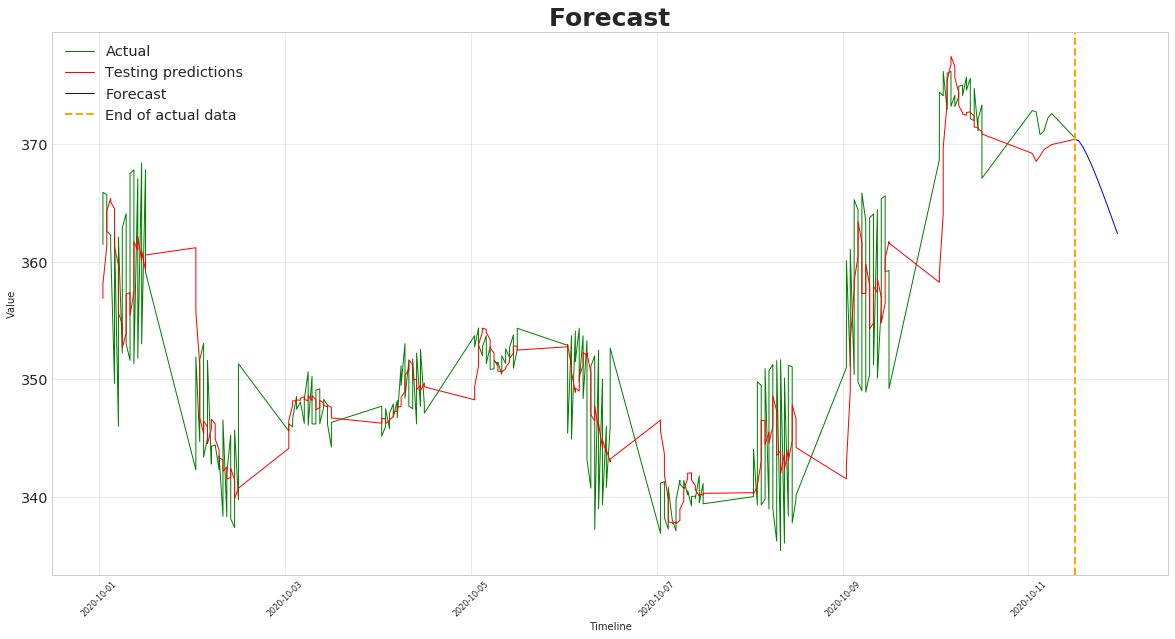# Predicting Ethereum (ETH) Prices With RNN-LSTM in Keras (TensorFlow)# Build LSTM network

It is now time to prepare the LSTM model, I define a function which takes as input the training and test data as well as some hyper parameters.

The model is then formed with two LSTM hidden layers, each with 50 units.

25% dropout layers are also used between each LSTM hidden layer.

A dropout on the input means that for a given probability, the data on the input connection to each LSTM block will be excluded from node activation and weight updates.

In Keras, this is specified with a dropout argument when creating an LSTM layer. The dropout value is a percentage between 0 (no dropout) and 1 (no connection).

It is important to specify the input shape on the first LSTM hidden layer so that it uses the same as the training data.

Linear activation is then used on the Dense output layer.

`def train_keras_model(X_train, y_train, X_test, y_test, epochs, batch_size, shuffle=False):  from tensorflow.keras.models import Sequential  from tensorflow.keras.layers import LSTM, Dropout, Dense# Initializing the Neural Network based on LSTM  model = Sequential()    model.add(LSTM(units=50,return_sequences=True,input_shape=(X_train.shape, X_train.shape)))  model.add(Dropout(0.25))  model.add(LSTM(units=50))  model.add(Dropout(0.25))  model.add(Dense(units=X_train.shape, activation='linear'))  model.compile(optimizer='adam',loss='mean_squared_error')  history = model.fit(X_train, y_train, shuffle=shuffle, validation_data=(X_test, y_test), epochs=epochs, verbose=2, batch_size=batch_size).history  return history, model`

The training can begin, I used 30 epochs with a batch size set to 256. These values seem to make the model converge quickly.

`# Fit modelhistory, model = train_keras_model(X_train, y_train, X_test, y_test, epochs=30, batch_size=256, shuffle=False)`

Here are the training and validation loss curves :

The model seems to converge quickly to 0 on both training and validation data.